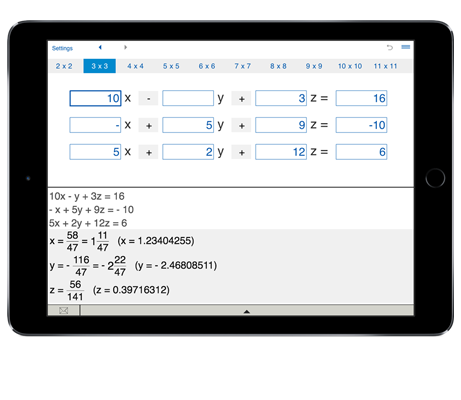# Solving systems of linear equations

This advanced system solver is intended for students, engineers and anyone who needs to solve systems of linear equations with up to 11 unknowns.
Solves 2x2, 3x3, 4x4, 5x5, 6x6, 7x7, 8x8, 9x9, 10x10 and 11x11 systems of linear equations.

## Systems of linear equations solver

Solving systems of linear equations with 3 and more variables is a difficult and time consuming task.

Systems of Linear Equations Solver is an advanced calculator that has everything you need to solve systems of multiple linear equations.

Systems of Linear Equations Solver saves your time and lets you solve systems of up to 11 equations - solving systems of linear equations 2x2, 3x3, 4x4, 5x5, 6x6, 7x7, 8x8, 9x9, 10x10, 11x11.

To solve a system of N linear equations in N variables, select the number of equations, enter the coefficients of linear equations, then click "Solve".

System of Linear Equations Solver helps you solve systems of linear equations involving 2, 3, 4, 5, 6, 7, 8, 9, 10 and 11 variables:

solving systems of two linear equations in two variables (2 x 2 System of Linear Equations Solver),

three linear equations in three variables (3 x 3 System of Linear Equations Solver),

systems of four linear equations in 4 variables (4 x 4 System of Linear Equations Solver),

systems of 5 linear equations in 5 variables (5 x 5 Systems of Linear Equations Solver),

systems of 6 linear equations in 6 variables (6 x 6 Systems of Linear Equations Solver),

system of 7 linear equations in 7 variables (7 x 7 Systems of Linear Equations Solver), and more.

To solve a system of linear equations, enter the number of equations, type in the coefficients of linear equations and tap Solve. You may enter positive, negative, or zero values.